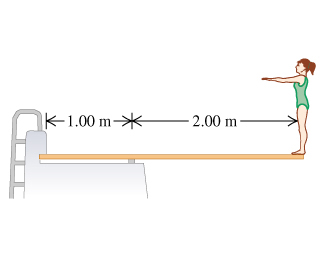# Problem: A diving board of length 3.00 m is supported at a point 1.00 m from the end, and a diver weighing 510 N stands at the free end. The diving board is of uniform cross-section and weighs 280 N.You may want to review (Pages 343 - 347).For related problemsolving tips and strategies, you may want to view a Video Tutor Solution of Locating your center of gravity while you work out.a. Find the magnitude of the force at the support point.b. Find the magnitude of the force at the left-hand end.c. Find the direction of the force at the support point.d. Find the direction of the force at the left-hand end.

###### FREE Expert Solution

The system is at equilibrium.

Net torque:

$\overline{){\mathbf{\Sigma }}{\mathbf{\tau }}{\mathbf{=}}{\mathbf{0}}}$

Torque:

$\overline{){\mathbf{\tau }}{\mathbf{=}}{\mathbf{r}}{\mathbf{F}}{\mathbf{s}}{\mathbf{i}}{\mathbf{n}}{\mathbf{\theta }}}$ where θ is the angle between r and F.

Therefore, the clockwise and counterclockwise moments are equal.

Let's take the force at the support point to be Fs and that at the left-hand side be FL. Let's assume the direction of these forces relative to the board is unknown.###### Problem DetailsA diving board of length 3.00 m is supported at a point 1.00 m from the end, and a diver weighing 510 N stands at the free end. The diving board is of uniform cross-section and weighs 280 N.

You may want to review (Pages 343 - 347).

For related problemsolving tips and strategies, you may want to view a Video Tutor Solution of Locating your center of gravity while you work out.

a. Find the magnitude of the force at the support point.

b. Find the magnitude of the force at the left-hand end.

c. Find the direction of the force at the support point.

d. Find the direction of the force at the left-hand end.